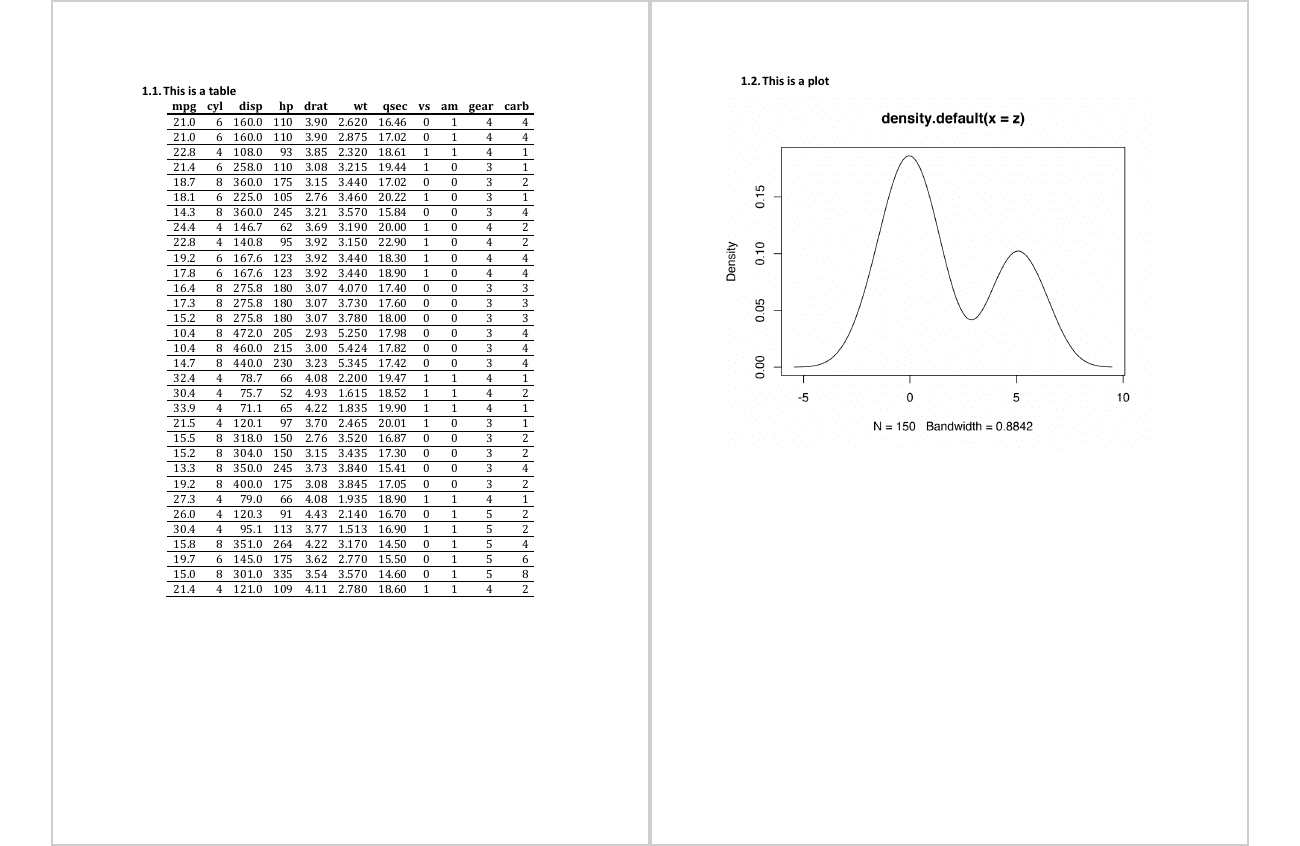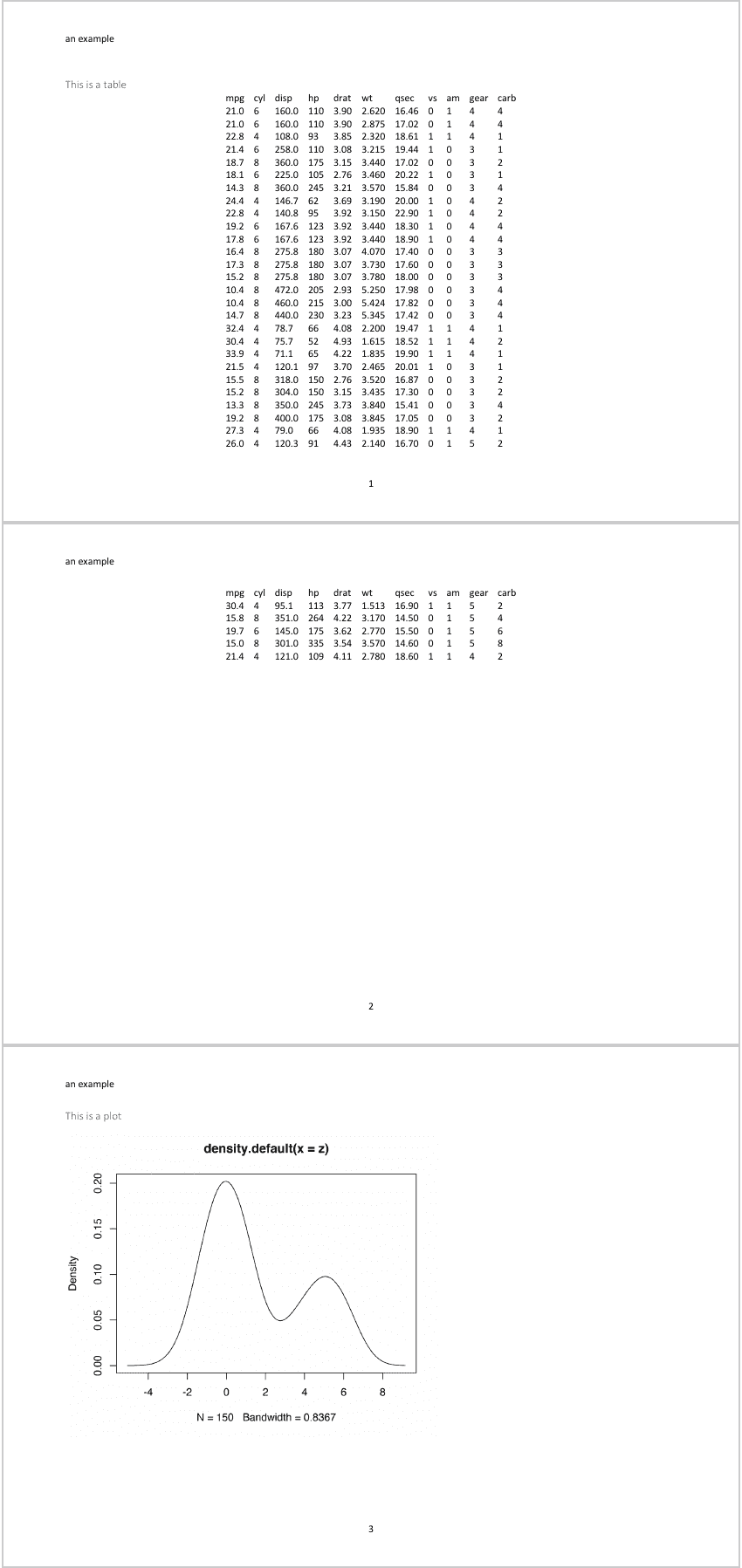read and import a docx file as an R object representing the document. When no file is specified, it uses a default empty file.

Use then this object to add content to it and create Word files from R.

read_docx(path = NULL)

# S3 method for rdocx
print(x, target = NULL, ...)

## Arguments

path

path to the docx file to use as base document.

x

an rdocx object

target

path to the docx file to write

...

unused

## Value

an object of class rdocx.

## Methods (by generic)

• print: write docx to a file. It returns the path of the result file.

## styles

read_docx() uses a Word file as the initial document. This is the original Word document from which the document layout, paragraph styles, or table styles come.

You will be able to add formatted text, change the paragraph style with the R api but also use the styles from the original document.

See body_add_* functions to add content.

## Illustrations## Examples

library(officer)

pinst <- plot_instr({
z <- c(rnorm(100), rnorm(50, mean = 5))
plot(density(z))
})

doc_1 <- body_add_par(doc_1, "This is a table", style = "heading 2")
doc_1 <- body_add_table(doc_1, value = mtcars, style = "table_template")
doc_1 <- body_add_par(doc_1, "This is a plot", style = "heading 2")
doc_1 <- body_add_plot(doc_1, pinst)
docx_file_1 <- print(doc_1, target = tempfile(fileext = ".docx"))

template <- system.file(package = "officer",
"doc_examples", "landscape.docx")
doc_2 <- read_docx(path = template)
doc_2 <- body_add_par(doc_2, "This is a table", style = "heading 2")
doc_2 <- body_add_table(doc_2, value = mtcars)
doc_2 <- body_add_par(doc_2, "This is a plot", style = "heading 2")
doc_2 <- body_add_plot(doc_2, pinst)
docx_file_2 <- print(doc_2, target = tempfile(fileext = ".docx"))